# Segmenting data with Segmentr

#### 2019-08-28

Segmentr is a package that implements a handful of algorithms to segment a given data set, by finding the change points that maximize the collective likelihood of the segments according to an arbitrary likelihood function. So, the user of this package has to find an adequate likelihood for the segmentation problem to be solved, possibly having to penalize it in order to avoid either an overparametrized or underparametrized model, i.e. one with too many or too few change points, respectively. Also, it’s important to consider the computation time of each algorithm and its trade-offs. This vignette walks rough the main concepts regarding its usage, using historical temperature data from Berlin as an example. For this demo, the following packages will be used:

require(segmentr)
require(tidyr)
require(tibble)
require(dplyr)
require(lubridate)
require(magrittr)
require(purrr)

## Understanding the data

The berlin data set, provided in this package, contains daily temperature measurements from 7 weather stations in Berlin for every day in the years 2010 and 2011, i.e., a total of 730 days. Therefore, every element in the data set has a temperature data point with units in Celsius, such that each of the 730 columns corresponds to a date, and each of the 7 rows corresponds to the weather station the data point was measured at. In the table below, it’s possible to see the first three columns, as well as the last three columns, together with the respective stations.

data(berlin)
as_tibble(berlin, rownames="station") %>%
mutate(..="..") %>%
select(station, 2010-01-01:2010-01-03, .., 2011-12-29:2011-12-31)
#> # A tibble: 7 x 8
#>   station 2010-01-01 2010-01-02 2010-01-03 ..    2011-12-29
#>   <chr>          <dbl>        <dbl>        <dbl> <chr>        <dbl>
#> 1 Berlin…         -1.6         -1.9         -4.8 ..             6
#> 2 Berlin…         -1.9         -2.3         -4.5 ..             5.2
#> 3 Berlin…         -1.9         -2           -4.6 ..             5.2
#> 4 Berlin…         -1.8         -1.9         -4.8 ..             5.7
#> 5 Berlin…         -1.9         -2.1         -4.6 ..             5
#> 6 Berlin…         -1.5         -2           -4.5 ..             6
#> 7 Berlin…         -1.4         -1.7         -4.3 ..             5.7
#> # … with 2 more variables: 2011-12-30 <dbl>, 2011-12-31 <dbl>

In order to grasp the behavior of the weather data, we plot the daily average temperature of all the weather stations, i.e., the mean value of each column in the data set as a time series graph in order to observe how the average temperature of Berlin behaves over time.

berlin %>%
colMeans() %>%
enframe("time", "temperature") %>%
mutate_at(vars(time), ymd) %>%
with(plot(time, temperature, cex=0.2))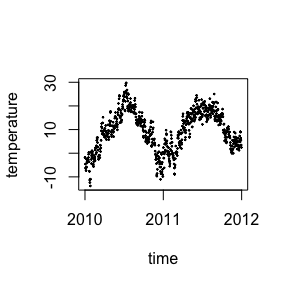In the graph, the daily temperatures points alternate in upwards and downwards trends, which suggests it’s possible to fit linear regressions for each of the upwards or downwards trend segments. So, a “linear regression likelihood” function is proposed, which should return a higher value the better a linear regression fits in a given segment. By intuition, we expect the likelihood function to identify segments approximately like presented below.

plot_results <- function(results, data) {
dates <- colnames(data) %>% ymd()

data %>%
colMeans() %>%
enframe("time", "temperature") %>%
mutate_at(vars(time), ymd) %>%
with({
plot(time, temperature, cex=0.2)
abline(v=dates[results$changepoints], col="red", lty=2) }) } plot_results(list(changepoints=c(200, 360, 570)), berlin)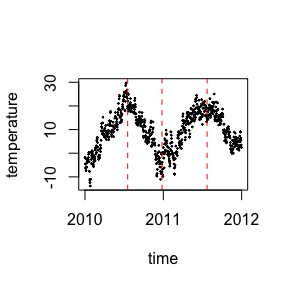## Building the likelihood An adequate likelihood function should be able to rank a given set of possible segments and pick the best one given the evaluation criteria. Since the goal is to select segments with a good linear regression fit, the method’s own standard log-likelihood function, i.e. the negative of the squared sum of residuals, is a good candidate for our segment likelihood function. However, the sum of residuals tend to increase with the amount of points in a segment. Instead, we pick the negative mean of the squared residuals, because it normalizes the likelihoods based on the length of the segment. So, in the equation, $$L_lm$$ is the linear regression likelihood function, $$X$$ is the set of points that belong to the segment, $$x_i$$ and $$y_i$$ are the points that belong to $$X$$ for each index $$i$$, and $$f$$ is the best linear regression that fitted the $$X$$ segment. $L_{lm}(X)=-\sum_{i=1}^n\frac{1}{n}(y_i - f(x_i))^2$ A [segment()] likelihood argument requires a function which accepts a candidate segment matrix, i.e. a subset of the columns in the data set, and returns the estimated likelihood for the candidate. Therefore, the lm_likelihood function implementation is provided below, one which obeys the contract by taking a matrix as argument and returning the negative mean of the squared residuals of a linear regression over the candidate segment. The likelihoods for a small, a medium, and a large segment are also provided, in order to compare the magnitude of likelihood values function for different sizes of segments. lm_likelihood <- function (data) { fit <- t(data) %>% as_tibble() %>% rowid_to_column() %>% gather(station, temperature, -rowid) %>% with(lm(temperature ~ rowid)) -mean(fit$residuals ^ 2)
}

c(lm_likelihood(berlin[, 2:3]), lm_likelihood(berlin[, 1:150]), lm_likelihood(berlin))
#>   -0.02836735 -12.18277010 -68.85184724

With the likelihood function defined, it can now be applied to [segment()] in order to get the segments for the data set. Since the time complexity of the exact algorithm is $$O(n^2)$$ and the number of points in the data set is high, the execution time required for the computation is quite prohibitive. So, for demonstration purposes, we use the hierarchical algorithm, due to its $$O(n \log(n))$$. We point the hierarchical algorithm, generally suitable for the [multivariate()] likelihood, assumes the segments’ likelihoods to be structured in a hierarchical manner, with a combination of two neighboring segments having being selected as an intermediate step before evaluating the ideal change points of the data set.

results <- segment(
berlin,
likelihood = lm_likelihood,
algorithm = "hierarchical"
)

results
#> Segments (total of 19):
#>
#> 1:2
#> 3:6
#> 7:17
#> 18:19
#> 20:21
#> 22:23
#> 24:24
#> 25:26
#> 27:28
#> 29:29
#> 30:31
#> 32:33
#> 34:35
#> 36:37
#> 38:38
#> 39:41
#> 42:43
#> 44:47
#> 48:730

With the results calculated, it’s possible to plot them in the together with the weather data.

plot_results(results, berlin)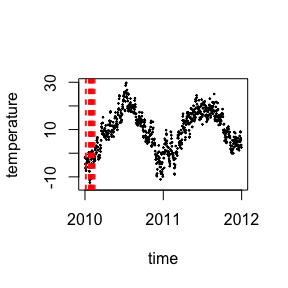From the segments computed using the bare lm_likelihood function, it’s possible see many very short segments, and a very large last segment. This is a result of the algorithm used, as well as the fact the lm_likelihood function tends to favor very short segments, as they usually have smaller residual error. So, to not get segments too short or too long, it’s necessary to penalize the likelihood function for either extremely short or extremely long lengths.

## Penalizing the likelihood function

To penalize a likelihood function in the Segmentr context is to decrease the return value of the likelihood function whenever unwanted segments are provided as an input. Typically, this involves penalizing the likelihood function whenever a very short or a very long segment is provided.

One such method is to subtract the output of the likelihood function with a penalty function which is a function of the length of the segment. We propose a penalty function.

$p(l) = C_1e^{s_1(l - \frac{L}{2})} + C_2e^{s_2(-l + \frac{L}{2})}$

The penalty $$p$$, function of the segment’s length $$l$$, has the property that, for parametrization values $$C_1 > 0$$, $$s_1 > 0$$, $$C_2 > 0$$ and $$s_2 > 0$$, the penalty is high for values of $$l$$ neighboring $$0$$, as well as values of $$l$$ the total length $$L$$ of the data set. However, penalty is close to its minimum for values of $$l$$ neighboring $$\frac{L}{2}$$. To visualize, consider a sample penalty function, with $$C_1 = C_2 = 1$$, $$s_1 = s_2 = 0.3$$ and $$L = 100$$, plotted below.

plot_curve <- function(expr, from, to, points = 100, plot_func=plot, ...) {
x <- floor(seq(from, to, length.out = 100))
y <- map_dbl(x, expr)
plot_func(x, y, ...)
}

plot_curve(~ exp(0.3*(. - 50)) + exp(0.3 * (-. + 50)), from = 0, to = 100, type="l")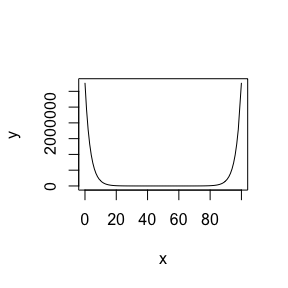Given the penalty function general formula, it’s necessary to adjust the parameters such the penalty function has a scale compatible with the likelihood function. The [auto_penalize()] function builds a penalized version of the likelihood function, by estimating parametrization values based on sample likelihood values for big and small segments of the data set provided. The estimated parameters are tunable by adjusting the small_segment_penalty and the big_segment_penalty, depending on how much small or big segments, respectively, should be penalized, i.e. the higher the parameter, the more penalized the related type of segment size is.

Let $$P_s$$ be the small_segment_penalty, $$P_b$$ be the big_segment_penalty, $$\mu_s$$ be the average likelihood for the sampled small segments and $$\mu_b$$ be the average likelihood for the samples big segments. The relationship between the parameters is defined in the equations below.

$C_1 = \frac{\mu_b}{P_b} \\ s_1 = \frac{4 \log(P_b)}{L} \\ C_2 = \frac{\mu_s}{P_s} \\ s_2 = \frac{4 \log(P_s)}{L}$

So, a penalized_likelihood function is created with [auto_penalize()] and then used with [segment()].

penalized_likelihood <- auto_penalize(berlin, lm_likelihood)
results <- segment(
berlin,
likelihood = penalized_likelihood,
algorithm = "hierarchical"
)
results
#> Segments (total of 6):
#>
#> 1:203
#> 204:268
#> 269:327
#> 328:559
#> 560:644
#> 645:730

With the results, it’s possible to the segments with the weather data.

plot_results(results, berlin)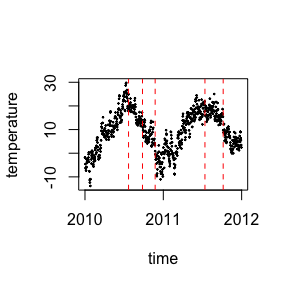The function above found two segments, the last of which was still quite large, which suggests the big_segment_penalty need to be increased to avoid that type of segment in the function.

penalized_likelihood <- auto_penalize(berlin, lm_likelihood, big_segment_penalty = 1000)
results <- segment(
berlin,
likelihood = penalized_likelihood,
algorithm = "hierarchical"
)
results
#> Segments (total of 4):
#>
#> 1:239
#> 240:327
#> 328:644
#> 645:730

Again, with the results, it’s possible to the segments with the weather data.

plot_results(results, berlin)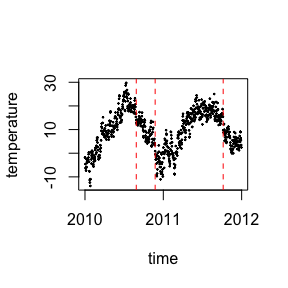Even with the new adjusted penalized_likelihood, the data set isn’t segmented into ideal segments. The reason behind this, as discusses earlier. So, in order to segment the data ideally, it’s necessary to evaluate all of the possibilities.

The exact algorithm does precisely compute all of the possibilities, but its $$O(n^2)$$ time complexity is quite prohibitive to run the computation on the entire data set. So we can actually make the computation time tolerable by reducing the granularity of the data, getting the monthly averages for each of the the measurement stations.

## Reducing granularity

The data set needs to be resampled in order to represent the monthly weather averages. It is done by computing the average temperature for each combination of month and weather station. With the granularity reduction, the data set will have 24 columns, one for each month in the two years period comprehended. The plot of the monthly average temperature of the temperatures of all the data points is plotted below.

monthly_berlin <- berlin %>%
as_tibble(rownames = "station") %>%
gather(time, temperature, -station) %>%
mutate(month = floor_date(ymd(time), "month")) %>%
group_by(station, month) %>%
summarize(temperature = mean(temperature)) %>%
stations <- .$station result <- as.matrix(.[, -1]) rownames(result) <- stations result } monthly_berlin %>% colMeans() %>% enframe("time", "temperature") %>% mutate_at(vars(time), ymd) %>% with(plot(time, temperature, cex=0.2))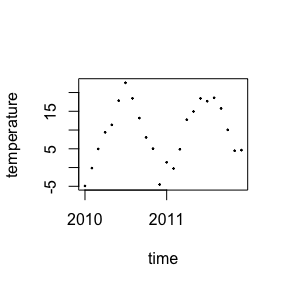A new penalized_function is then built and applied to the monthly data set using [segment()]. penalized_likelihood <- auto_penalize(monthly_berlin, lm_likelihood, small_segment_penalty = 100) results <- segment( monthly_berlin, likelihood = penalized_likelihood, algorithm = "exact" ) results #> Segments (total of 4): #> #> 1:6 #> 7:11 #> 12:18 #> 19:24 With the results of the monthly data segmentation, we plot it with the weather data. plot_results(results, monthly_berlin)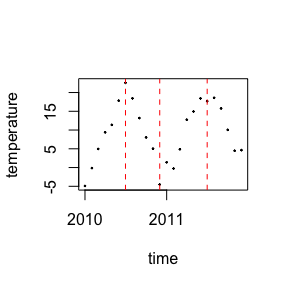With the exact solution, made possible with the granularity reduction, we noticed the data set is segmented closer to what the ideal solution would be, as the algorithm as able to evaluate all of the possibilities. ## A non-usual likelihood function Take notice the picking of the likelihood just have be able to rank segments in a desired manner. Therefore, there’s freedom to pick a non-conventional likelihood function. For example, the R-squared statistic of the linear regression in each segment is conventionally used to infer how well the linear model fits the points in the data. It ranges from zero to one and the closer it is to one, the better it predicts the points in the data. Therefore, a rsquared_likelihood is defined and it can be used to figure what segments better fit linear regressions. rsquared_likelihood <- function (data) { as_tibble(t(data)) %>% rowid_to_column() %>% gather(station, temperature, -rowid) %>% with(lm(temperature ~ rowid)) %>% summary %>% .$adj.r.squared
}

c(rsquared_likelihood(berlin[, 2:3]), rsquared_likelihood(berlin[, 1:150]), rsquared_likelihood(berlin))
#>  0.98211599 0.76014938 0.03283039

Similar to the previous case, the new rsquared_likelihood has the highest values for small segments. Therefore, it needs to be penalized with the auto_penalize function.

penalized_likelihood <- auto_penalize(berlin, rsquared_likelihood)
results <- segment(
berlin,
likelihood = penalized_likelihood,
algorithm = "hierarchical"
)
results
#> Segments (total of 3):
#>
#> 1:268
#> 269:550
#> 551:730

With the results, we plot it with the weather data.

plot_results(results, berlin)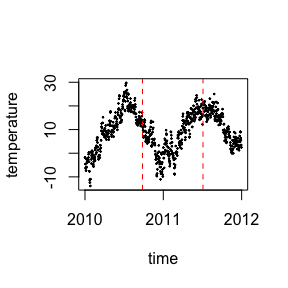The default penalized rsquared_likelihood split the data set in three segments, reasonably well distributed. Since the penalty model applied by auto_penalize apply the least penalty for values segments closer to about half the total length, increasing the big_penalty_segment will have no effect. In fact, smaller segments are being over penalized. Because of this, it’s necessary to reduce the small_segment_penalty segment.

penalized_likelihood <- auto_penalize(berlin, rsquared_likelihood, small_segment_penalty = 1.5)
results <- segment(
berlin,
likelihood = penalized_likelihood,
algorithm = "hierarchical"
)
results
#> Segments (total of 6):
#>
#> 1:237
#> 238:364
#> 365:366
#> 367:587
#> 588:724
#> 725:730

With the r_squared_likelihood results, we plot it with the weather data.

plot_results(results, berlin)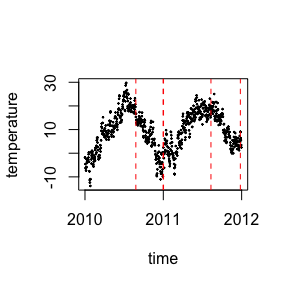With the adjusted parameters, the new penalized rsquared_likelihood was able to segment the data in a more accurate manner, despite the nature of the hierarchical algorithm. As discussed previously, we point the hierarchical algorithm is not adequate for the linear likelihood, as the grouping of neighboring segments under a macro-segment, an intermediate segment state under the hierarchical algorithm, has a unattractive likelihood, due to the presence of alternating trends. Because of this, the macro-segment is never picked by the algorithm during the computation process, and so the ideal change points are never picked at their ideal positions. In order to see it more clearly, take the first 547 data points of the Berlin data set, roughly one year and a half.

sub_berlin <- berlin[, 1:547]
penalized_likelihood <- auto_penalize(sub_berlin, rsquared_likelihood)
results <- segment(
sub_berlin,
likelihood = penalized_likelihood,
algorithm = "hierarchical"
)
results
#> Segments (total of 3):
#>
#> 1:178
#> 179:282
#> 283:547

With the penalized_likelihood results, we plot it with weather data.

plot_results(results, sub_berlin)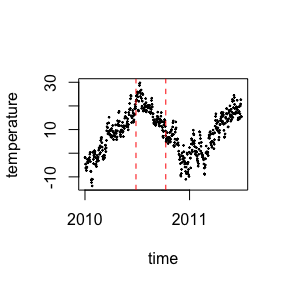From the results, we can clearly see the segments do not match our expectations, as the algorithm is not able to bisect a macro-segment before finding the ideal segments in the next algorithm iteration.